The analysis of trusses

A truss: A truss is a structure made of two force members all pin connected to each other.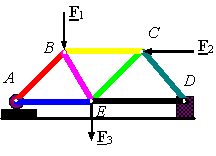The method of joints: This method uses the free-body-diagram of joints in the structure to determine the  forces in each member. For example, in the above structure we have 5 joints each having a free body diagram as follows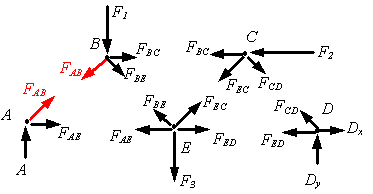Note how Newton’s third law controls how one introduces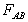on the joints A and on the joint B. For each joint one can write two equations (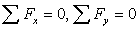). The moment equation is trivially satisfied since all forces on a joint pass trough the joint. For example, for the above truss we have 5 joints, therefore we can write 10 equations of equilibrium (two for each joint). In the above example there are seven unknown member forces (FAB, FBC, FCD, FED, FEC, FBE, FAE)  plus three unknown support reactions (A, Dx, Dy), giving a total of 10 unknowns to solve for using the 10 equations obtained from equilibrium.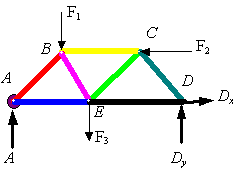The method of sections: This method uses free-body-diagrams of sections of the truss to obtain unknown forces. For example, if one needs only to find the force in BC, it is possible to do this by only writing two equations. First, draw the free body diagram of the full truss and solve for the reaction at A by taking moments about D. Next draw the free body diagram of the section shown and take moments about E to find the force in BC.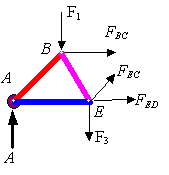In the method of sections one can write three equations for each free-body-diagram (two components of force and one moment equation).

Things to consider:

·        Zero force members: Some members in a truss cannot carry load. These members are called zero force members. Examples of zero force members are the colored members (AB, BC, and DG) in the following truss.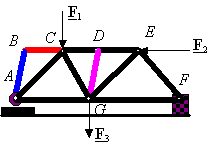Consider the following free-body-diagrams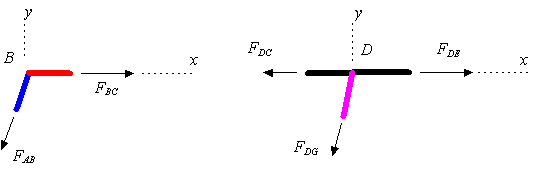If you sum the forces in the y-direction in the left free-body-diagram, you will see that FAB must be zero since it is not balanced by another force. Then if you sum forces in the y-direction you will find that FBC must also be zero. If you sum the forces in the y direction in the right free-body-diagram, you will see that FDG must be zero since it is not balanced by another force.

·        A redundant joint: Sometimes a joint is redundant. For example, in the following free-body-diagram the load is directly transmitted from each member to the one opposite it without any interaction.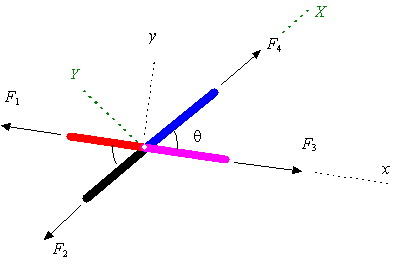By summing forces along the y-direction one will get F2=F4, and by summing forces along the Y‑direction one will get F1=F3.

·        Redundant members: Sometimes a structure contains one or more redundant members. These members must be removed from the truss, otherwise one will have an insufficient number of equations  to solve for the unknown member forces. Slender members are not very useful in compression since they buckle and, as a result, lose their load carrying capability. For example, in the following truss one of the two members AC or BD is redundant. To solve the problem, we remove member BD which will go into compression as a result of the applied loading (i.e., the diagonal AC will have to increase in length and the diagonal BD will have to decease in length for the structure to bend to the right). If we did not remove this member we would have 9 unknowns (five member loads and four support reactions) and only 8 equations (two for each joint).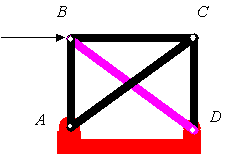·        Mechanisms: Sometimes there is too much freedom in a structure. For example, the following structure cannot carry any load since it will collapse under the load.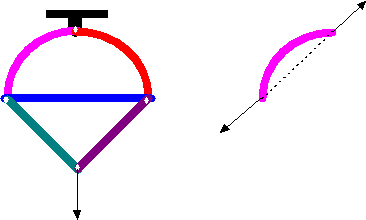·        Curved members: Remember that the two forces acting on a two-force-member are along the line connecting the two points on which the loads are applied.